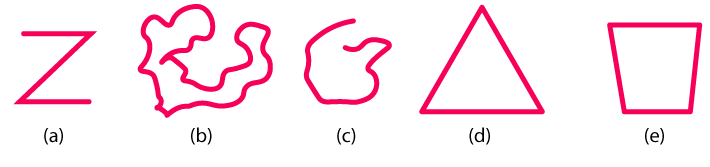# Classify the following curves as (i) Open or (ii) ClosedSolution

(a) The given curve is an open curve

(b) The given curve is closed curve

(c) The given curve is open curve

(d) The given curve is closed curve

(e) The given curve is closed curve(0)(0)